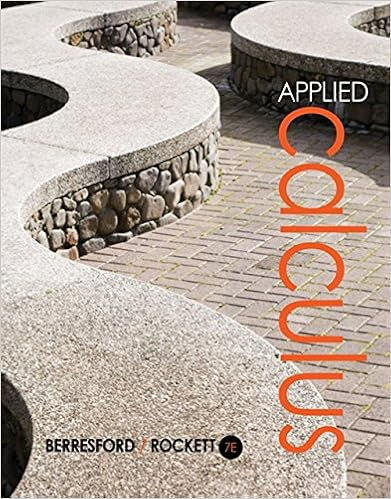# 4 x 12 original equation 4 x 4 12 4 subtract 4 from

• 97

This preview shows page 83 - 86 out of 97 pages.

##### We have textbook solutions for you!
The document you are viewing contains questions related to this textbook.The document you are viewing contains questions related to this textbook.
Chapter 1 / Exercise 18
Applied Calculus
Berresford/RockettExpert Verified
4 + x = 12 Original equation. 4 + x 4 = 12 4 Subtract 4 from both sides of the equation. x = 8 On the left, subtracting 4 “undoes” the effect of adding 4 and returns x . On the right, 12 4 = 8. 4. Answer the Question. The number is 8. 5. Look Back. Does the solution 8 satisfy the words in the original problem? We were told that “four more than a certain number is 12.” Well, four more than 8 is 12, so our solution is correct. Answer: 7 You Try It! EXAMPLE 8. Amelie withdraws \$125 from her savings account. Because of Fred withdraws \$230 from his account, lowering his balance to \$3,500. What was his original balance? the withdrawal, the current balance in her account is now \$1,200. What was the original balance in the account before the withdrawal? Solution. In our solution, we will carefully address each step of the Require- ments for Word Problem Solutions .
##### We have textbook solutions for you!
The document you are viewing contains questions related to this textbook.The document you are viewing contains questions related to this textbook.
Chapter 1 / Exercise 18
Applied Calculus
Berresford/RockettExpert Verified
1.6. SOLVING EQUATIONS BY ADDITION AND SUBTRACTION 83 1. Set up a Variable Dictionary. We can satisfy this requirement by simply stating “Let B represent the original balance in Amelie’s account.” 2. Set up an Equation. We can describe the situation in words and symbols. Original Balance minus Amelie’s Withdrawal is Current Balance B 125 = 1200 3. Solve the Equation . To “undo” the subtraction, add 125 to both sides of the equation. B 125 = 1200 Original equation. B 125 + 125 = 1200 + 125 Add 125 to both sides of the equation. B = 1325 On the left, adding 125 “undoes” the effect of subtracting 125 and returns B . On the right, 1200+125=1325. 4. Answer the Question. The original balance was \$1,325. 5. Look Back. Does the solution \$1,325 satisfy the words in the original problem? Note that if Amelie withdraws \$125 from this balance, the new balance will be \$1,200. Hence, the solution is correct. Answer: \$3,730. You Try It! EXAMPLE 9. The perimeter of a triangle is 114 feet. Two of the sides of The perimeter of a quadrilater is 200 meters. If three of the sides measure 20, 40, and 60 meters, what is the length of the fourth side? the triangle measure 30 feet and 40 feet, respectively. Find the measure of the third side of the triangle. Solution. In our solution, we will carefully address each step of the Require- ments for Word Problem Solutions. 1. Set up a Variable Dictionary. When geometry is involved, we can cre- ate our variable dictionary by labeling a carefully constructed diagram. With this thought in mind, we draw a triangle, then label its known and unknown sides and its perimeter.
84 CHAPTER 1. THE WHOLE NUMBERS x 30 ft 40 ft Perimeter = 114 ft The figure makes it clear that x represents the length of the unknown side of the triangle. The figure also summarizes information needed for the solution. 2. Set up an equation. We know that the perimeter of a triangle is found by finding the sum of its three sides; in words and symbols, Perimeter is First Side plus Second Side plus Third Side 114 = x + 30 + 40 Simplify the right-hand side by adding 30 and 40; i.e., 30 + 40 = 70.
•••